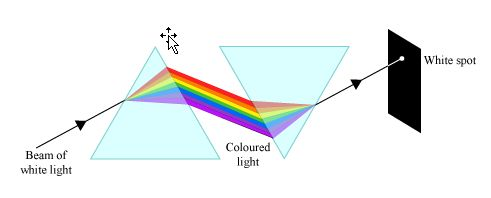Q

# Please help! A thin prism having refracting angle 10° is made of glass of refractive index 1.42. This prism is combined with another thin prism of glass of refractive index 1.7. This combination produces dispersion without deviation. The refracti

A thin prism having refracting angle 10° is made of glass of refractive index 1.42. This prism is combined with another thin prism of glass of refractive index 1.7. This combination produces dispersion without deviation. The refracting angle of second prism should be :

• Option 1)

• Option 2)

• Option 3)

• Option 4)

10°

537 Views

As we learnt in

Condition for deviation without dispersion -- wherein

= Refractive index of violet ( prism 1)

Refractive index of red ( prism 1)

= Refractive index of violet ( prism 2)

Refractive index of red ( prism 2)

For dispersion without deviation

Option 1)

This option is incorrect

Option 2)

This option is correct

Option 3)

This option is incorrect

Option 4)

10°

This option is incorrect

Exams
Articles
Questions